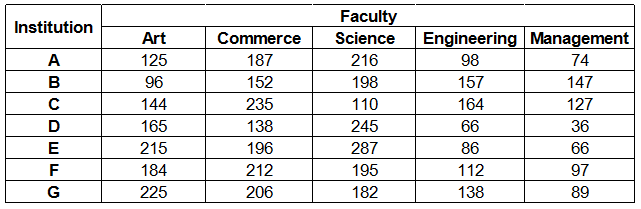### IBPS Clerk 2018 Question 18

Instructions

Study the following table carefully and answer the questions given below :
Number of students studying in different faculties in Seven institutionsQuestion 18

# The total number of students studying Art in institutes A, B and C together is approximately what per cent of total number of students studying Commerce in institutes D, E, F and G together ?

Solution

Total number of students studying Art in institutes A, B and C together

= 125 + 96 + 144 = 365

Total number of students studying Commerce in institutes D, E, F and G together

= 138 + 196 + 212 + 206 = 752

=> Required % = $$\frac{365}{752} \times 100 = 48.5 \%$$

$$\approx 50 \%$$